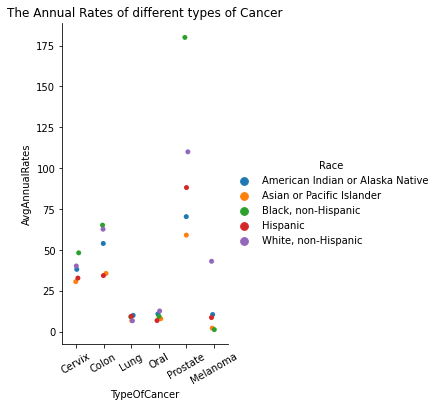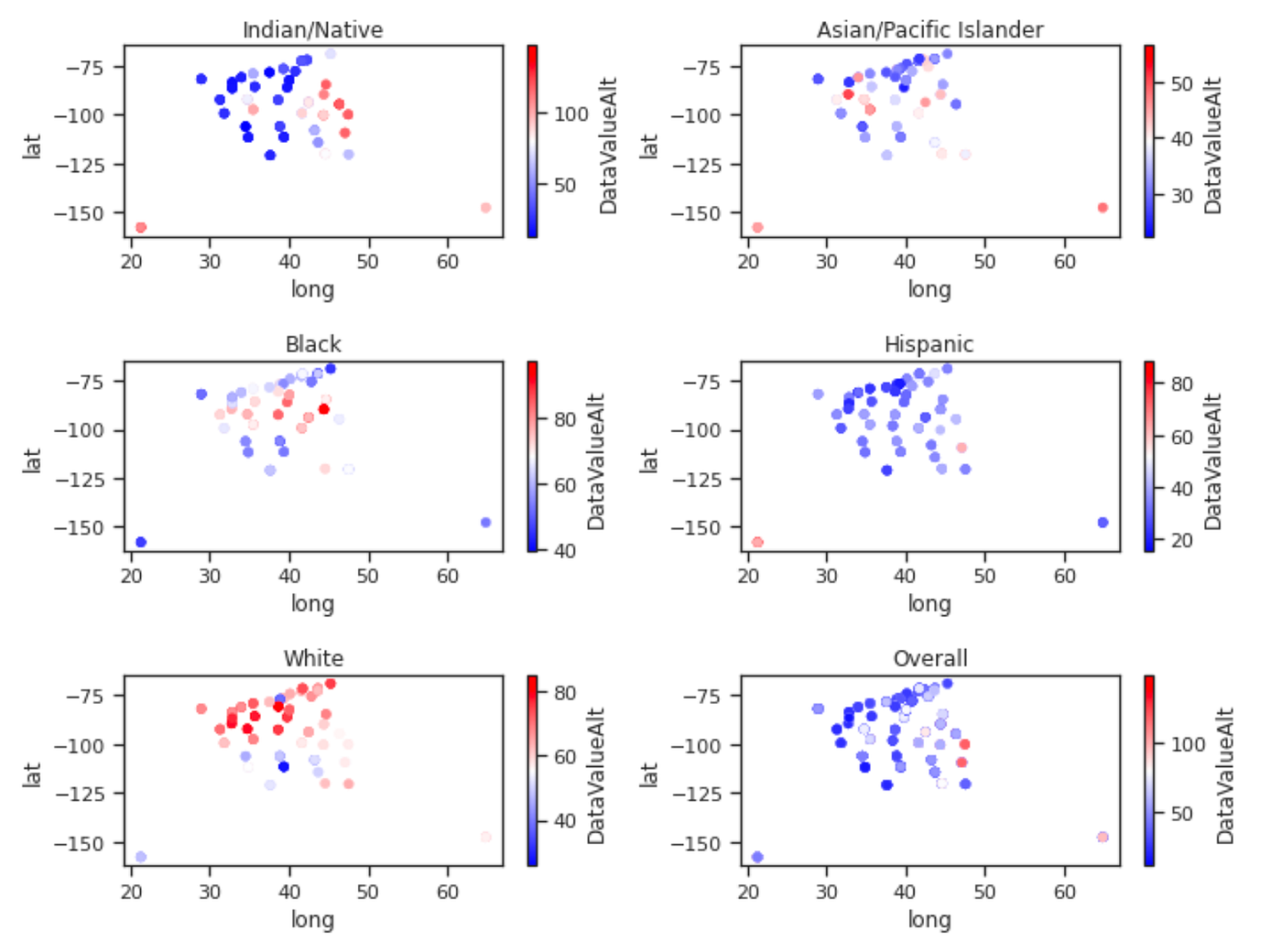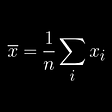# Multiple Hypothesis Testing: The average annual rates of lung cancer and patients’ races

## 4. EDAFigure 1: The discrepancy between annual rates of different cancer incidents of 5 racial groups in interestFigure 2: The geographic distribution of lung cancer rates among 5 racial groups in interest

## 5. Multiple Hypothesis Testing

• H0: Ri = Rj , with i and i representing 2 different races
• H1: Ri ≠ Rj
`def obtain_pvals(m, var, n): p_vals = np.array([]) for i in np.arange(5): for j in np.arange(i+1, 5): t = (m[i] — m[j]) / np.sqrt(var[i]/n[i] + var[j]/n[j]) df = n[i] + n[j] — 2 p = 2*(1 — stats.t.cdf(abs(t),df=df)) p_vals = np.append(p_vals,p) p_vals = p_vals[~np. isnan(p_vals)] return p_valsp_values = obtain_pvals(race.AvgAnnualRates, race.VarAnnualRates, race.n)`
• With Bonferroni adjustment, I simply reject any hypothesis with
P-value ≤ 0.05/10 = 0. 005.
`def bonferroni(p_values, alpha = 0.05):    n = len(p_values)    decisions =  p_values <= alpha/n    return decisionsbon = bonferroni(p_values)`
• On the other hand, to control FDR at level δ = 0.05, I will use the Benjamini-Hochberg Procedure as described below:
1. Order the unadjusted p-values: p1 ≤ p2 ≤ … ≤ p10
2. Then find the test with the highest rank j, for which:
`def benjamini_hochberg(p_values, alpha = 0.05):    n = len(p_values)    sorted_p = np.sort(p_values)        max_k = max([k for k in range(n) if sorted_p[k]<=(k + 1)*(alpha/n)])    threshold = sorted_p[max_k]decisions = p_values <= threshold    return decisionsbh = benjamini_hochberg(p_values)`

--

--

--

## More from Callie Nguyen

Love podcasts or audiobooks? Learn on the go with our new app.

## What’s the average IQ of Trump voters?## Notes | Understanding and Visualizing Data with Python: Week 2## Interactive NBA Draft Analysis## Misinterpretation of Data## Exploratory Data Analysis (EDA): A Practical Guide and Template for Structured Data## VIX: Wall Street’s “Fear Gauge”## Locate your Data and Boost it with Geo Processing## Google QUEST Q&A Labeling: 13th place solution## Introduction to the Measures of Central Tendency and Dispersion## Things you should know about x̄